Because I had some hard hours to figure this one out (basically I mixed a lot of degree and radians and messed up several times with tan and sin), here is my solution. It may be a help for someone (or for me later...).

The quest was to find the angle of a line through a point lying on a circle, when this line is parallel to some line passing through another point on the circle.

So let's get this started: Lets say, we got a point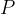with the coordinates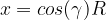and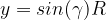, thus they are on the circle with radius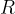, centered at the origin.

Now, we construct a line through the circles' circumference at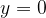, thus we know it must pass through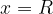. The line equation is then given by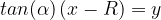.

Now, we construct a parallel line to it (in direction of negative x) with distance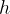, which is given by the line equation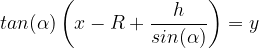.

To find now the angle, we insert the point into the equation, as we require that the line passes through this point: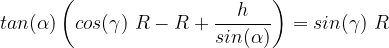.

I tried to solve this by hand but the formular we get is quite complex (and I found it using wolframalpha, not by hand).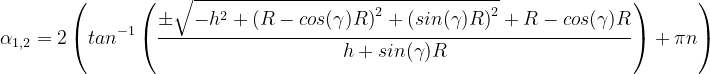with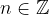. But as we know, that the angle must be between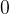and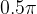, we can just set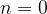and remove that part.

You might ask: why do you need something? Here is an example: lampe.scad I tried to arrange the rectangles in such a way, that they are rotated and touch each other. We can calculate now, that the angle (in this specific configuration) is about 57 degree.

Thank's to OpenSCAD, where you learn math the hard way ;)